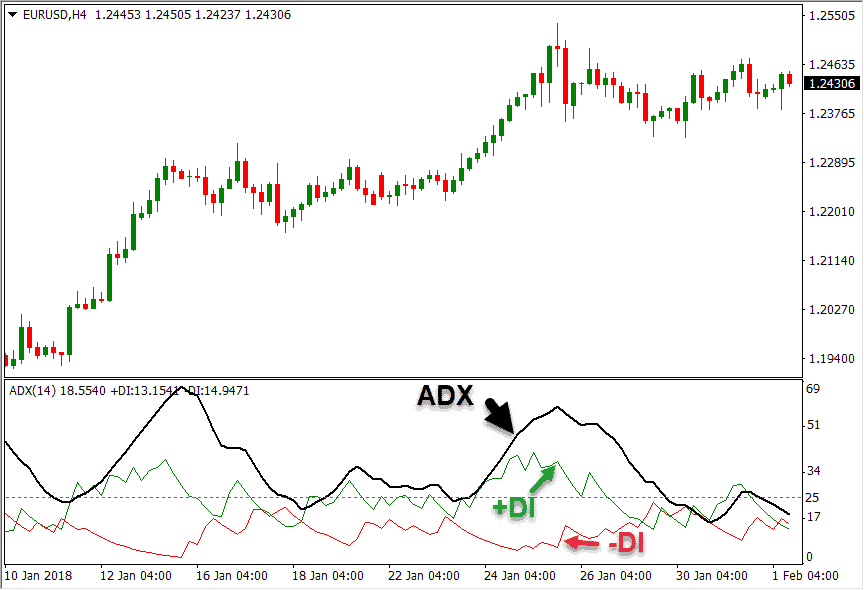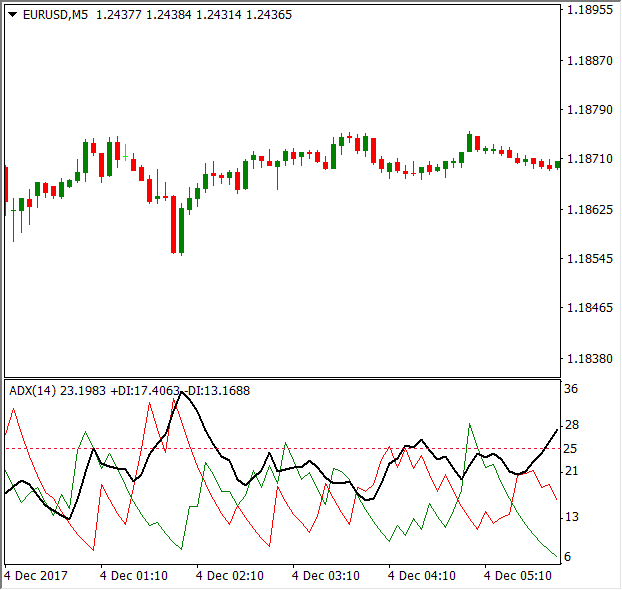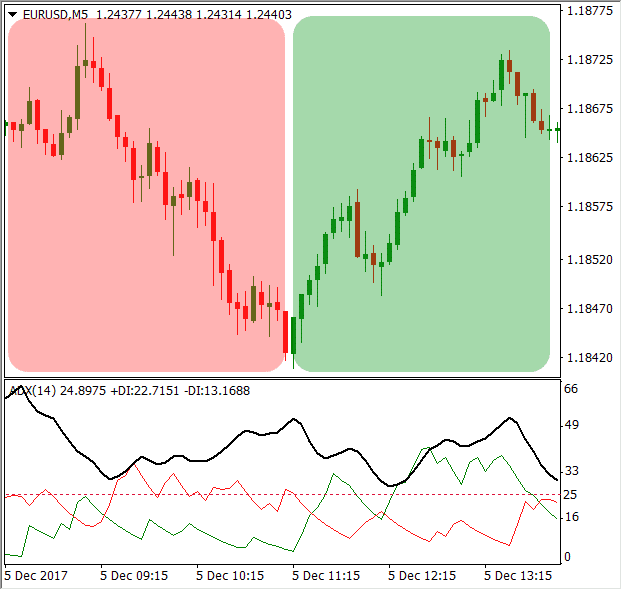# What is the ADX (Average Directional Movement Index) IndicatorWhat is the ADX (Average Directional Movement Index) Indicator
February 6, 2018

The ADX, or average directional movement index, is a technical indicator that measures the strength of the underlying trend. The indicator was developed by Welles Wilder for use in the commodities market, but it can also be used with stocks, currencies, futures or any other asset class.

It’s usually plotted below the price chart in a separate indicator window and includes two more lines called the DMI – directional movement indicators – on the basis of which the ADX indicator is calculated. It’s important to note that the ADX is non-directional, which means that it doesn’t measure the direction of the trend but only its strength. If you already have a good understanding of the ADX indicator and want to check a successful strategy for using it check out this post here.

The calculation of the ADX indicator is based on the calculation of the Plus Directional Movement (+DM), and Minus Directional Movement (-DM). The directional movement is positive in case when the current high minus the previous high is greater than the previous low minus the current low. This leads to a value of the +DM which equals the current high – previous high (if the result is negative, -DM equals 0).

On the other hand, a negative directional movement occurs when the current high minus the previous high is smaller than the previous low minus the current low. In this case, the -DM equals to the previous low – current low (if the result is negative, -DM equals 0).After the +DM and -DM values are calculated, they need to be smoothed out with the 14-day smoothed True Range. This is done by dividing both +DM and -DM with the 14-day smoothed True Range, to get the 14-day Minus Directional Indicator (-DI14) and 14-day Plus Directional Indicator (+DI14). Those are the two lines that are plotted along the ADX line.

The Directional Movement Index is then calculated as the absolute value of (+DI14 plus -DI14) / (+DI14 plus -DI14). Finally, the Average Directional Movement Index (ADX) is simply the 14-day smoothed average of the Directional Movement Index.

Basically, the ADX indicator can be used to identify if a currency pair is trending or not. The ADX doesn’t provide clues about the direction of the trend, but only about its strength. To measure the direction, the directional movement lines are used – if +DI is greater than -DI, an uptrend is intact, while a -DI reading larger than +DI indicates a downtrend.In addition, traders use the value of the ADX to determine whether to use a trend-following trading strategy or not. An ADX value below 25 represents a ranging market or a very weak trend. A value between 25 and 50 indicates a strong trend, values between 50 and 75 very strong trends, and values between 75 and 100 extremely strong trends.

The following picture shows a market with a very low ADX reading, which can be described as range-bound.The ADX spent the most time below the level of 25, indicating a ranging market or very weak trend. On the other hand, the following picture shows how the ADX behaves in times of very strong trends.The ADX indicator is above 25, ranging between values of 30 and 50 most of the time. This indicates a strong trend, but the ADX alone doesn’t reveal the direction of the trend. To identify if it’s a downtrend or uptrend, traders need to look on the Directional Movement lines for clues. As the chart above shows, the red -DI line is above the green +DI line during downtrend, and the +DI line is above the -DI line during uptrends.SummaryArticle Name
What is the ADX (Average Directional Movement Index) Indicator
Description
The ADX, or average directional movement index, is a technical indicator that measures the strength of the underlying trend. It's usually plotted below the price chart in a separate indicator window and includes two more lines called the DMI – directional movement indicators – on the basis of which the ADX indicator is calculated.
Author
Publisher Name